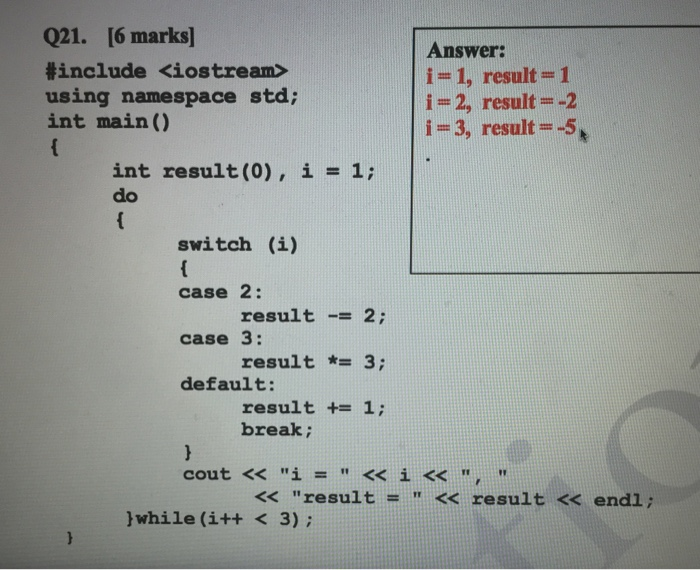# Answered! #include using namespace std: int main() { i = 1, int result(0), i = 1: do { switch (i) case 2: result -= 2: case 3: result *= 3: default: result += 1: break: } cout…

Please explain how this code resulted in the answers in DETAIL (C++)#include using namespace std: int main() { i = 1, int result(0), i = 1: do { switch (i) case 2: result -= 2: case 3: result *= 3: default: result += 1: break: } cout

The form of the do statement is

do
<statement>
while (<boolean_expression>);

first statement is executed.
then the boolean_expression is evaluated. If it
evaluates to true, statement is executed again.
this repetition continues until the
boolean_expression evaluates to false.

Don't use plagiarized sources. Get Your Custom Essay on
Answered! #include using namespace std: int main() { i = 1, int result(0), i = 1: do { switch (i) case 2: result -= 2: case 3: result *= 3: default: result += 1: break: } cout…
GET AN ESSAY WRITTEN FOR YOU FROM AS LOW AS \$13/PAGE

more explanation for do-while loop:

the codes inside the body of loop is executed at least once.then, only the test expression is checked.
if the test expression is true the body of loop is executed.this process continues until the test expression becomes false.
when the test expression is false do…while loop is terminated.

case 1:
in first case we have result=0 and i=1

now the controller comes first to the switch(i.e swithch(0)) statement initially with i=1 then switch becomes switch(1).
in our program we dont have case 1 so,the controller direcctly goes default case and executes it

result+=1

so,initally result value=0 and now 0+1=1
so now result =1 and i=1

as we observer after this statement result+=1 we have break statement now the controller print s i = 1 and result = 1.now controller enters into the while statement(i,e while(i++ < 3)

now i becomes 1 and it is lessthan 3 so,contion true again the controller enters into do loop with i=2 .

case 2:

in second case we have result=1 and i=1

now the controller comes first to the switch(i.e swithch(2)) statement with i=1 then switch becomes switch(2).
in our program we have case 2 so,the controller directly goes case 2 and executes it

result-=2

so, result value=1 and now 1-2=-1
so now result =-1

now the controller executes case 3 because after case 2 we dont have break statement

-1*3=-3

now the result = -3

now the controller executes default case because after case 3 we dont have break statement

-3 + 1 = -2

so after case 2 i=2 and result = -2

as we observer after this statement result+=1 we have break statement now the controller prints i = 1 and result = 1.now controller enters into the while statement(i,e while(i++ < 3)

now i becomes 2 and it is lessthan 3 so,contion true again the controller enters into do loop with i=2 .

case 3:the above step is repaeted so,

i=3 and result=-5